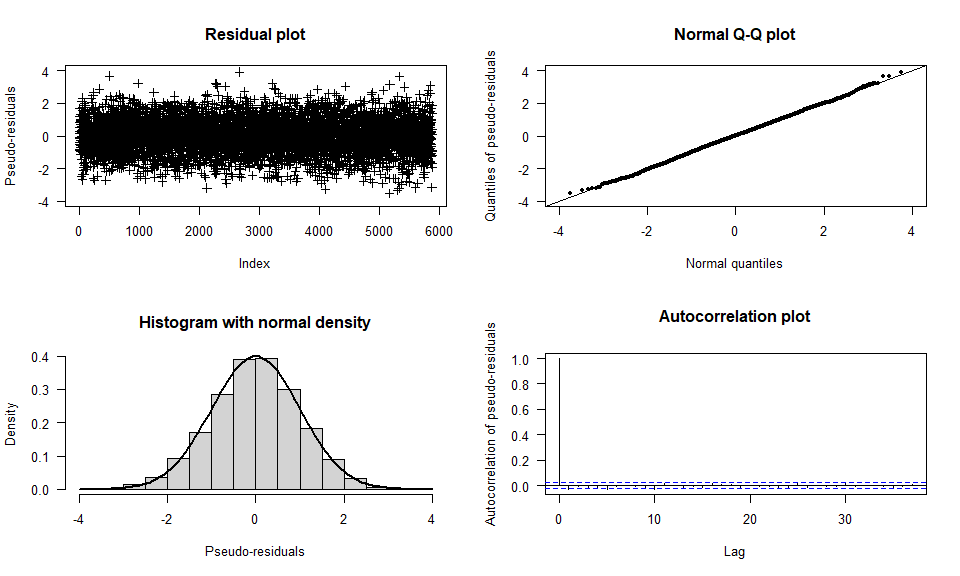# Model checking

This vignette1 discuss model checking in {fHMM}.

## Model checking using pseudo-residuals

Analyzing pseudo-residuals allows us to check whether the fitted model describes the data well. Since the observations are explained by different distributions (depending on the active state), this cannot be done by analyzing standard residuals. To transform all observations on a common scale, we proceed as follows: If $$X_t$$ has the invertible distribution function $$F_{X_t}$$, then

\begin{align*} Z_t=\Phi^{-1}(F_{X_t} (X_t)) \end{align*}

is standard normally distributed, where $$\Phi$$ denotes the cumulative distribution function of the standard normal distribution. The observations, $$(X_t)_t$$, are modeled well if the so-called pseudo-residuals, $$(Z_t)_t$$, are approximately standard normally distributed, which can be visually assessed using quantile-quantile plots or further investigated using statistical tests such as the Jarque-Bera test .

For HHMMs, we first decode the coarse-scale state process using the Viterbi algorithm. Subsequently, we assign each coarse-scale observation its distribution function under the fitted model and perform the transformation described above. Using the Viterbi-decoded coarse-scale states, we then treat the fine-scale observations analogously.

## Implementation

In {fHMM}, pseudo-residuals can be computed via the compute_residuals() function, provided that the states have been decoded beforehand.

We revisit the DAX example:

data(dax_model_3t)

The following line computes the residuals and saves them into the model object:

dax_model_3t <- compute_residuals(dax_model_3t)
#> Computed residuals

The residuals can be visualized as follows:

plot(dax_model_3t, plot_type = "pr")For additional normality tests, the residuals can be extracted from the model object via the residuals() method. The following lines exemplary perform a Jarque–Bera test :

tseries::jarque.bera.test(residuals(dax_model_3t))
#>
#>  Jarque Bera Test
#>
#> data:  residuals(dax_model_3t)
#> X-squared = 2.3625, df = 2, p-value = 0.3069

1. This vignette was build using R 4.2.2 with the {fHMM} 1.1.1 package.↩︎